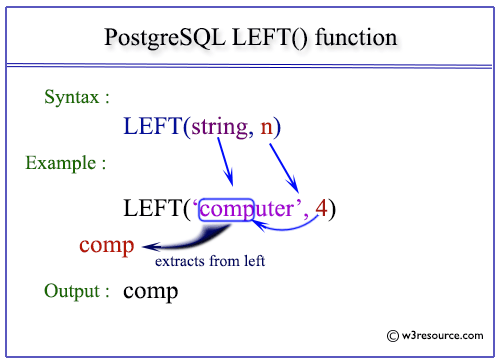# PostgreSQL LEFT() function

## LEFT() function

The PostgreSQL left() function is used to extract n number of characters specified in the argument from the left of a given string. When the value of n is negative, the extraction will happen from left except last n characters.

Syntax:

`left(string,n) `

PostgreSQL Version: 9.3

Pictorial Presentation of PostgreSQL LEFT() functionExample: PostgreSQL LEFT() function:

In the example below, the left function extracted 3 characters from the left of the string 'w3resource'.

Code:

``````SELECT left('w3resource',3)
AS "Extract 3 characters from the left";
```
```

Sample Output:

``` Extract 3 characters from the left
------------------------------------
w3r
(1 row)
```

Example: PostgreSQL LEFT() function using negative value:

In the example below, the left function extracted all the characters from the left side except 3 rightmost characters from the string 'w3resource', because the value of extracting character number is negative.

Code:

``````SELECT left('w3resource',-3)
AS "Extract all characters except 3 rightmost";
```
```

Sample Output:

``` Extract all characters except 3 rightmost
-------------------------------------------
w3resou
(1 row)
```

Example: PostgreSQL LEFT() function using columns of table:

Sample Table: employees

If we want to display the first_name, last_name for those employees whose first 3 characters of their name contain 'Nan' from employees table, the following sql statement can be used:

Code:

``````SELECT first_name, last_name
FROM employees
WHERE left(first_name,3)='Nan';
```
```

Sample Output:

``` first_name | last_name
------------+-----------
Nancy      | Greenberg
Nanette    | Cambrault
Nandita    | Sarchand
(3 rows)
```
﻿

New Content: Composer: Dependency manager for PHP, R Programming